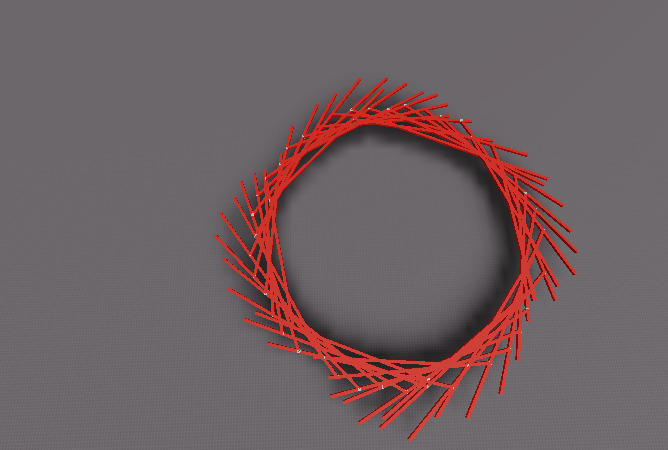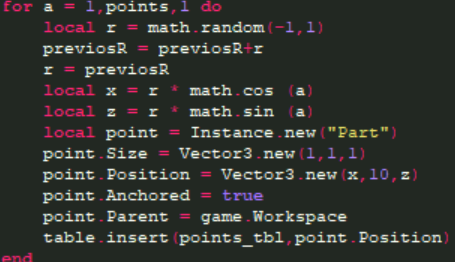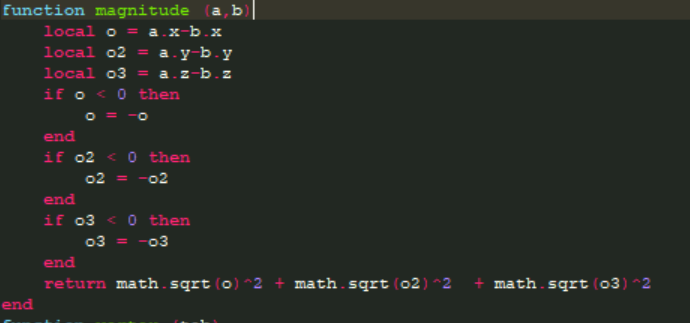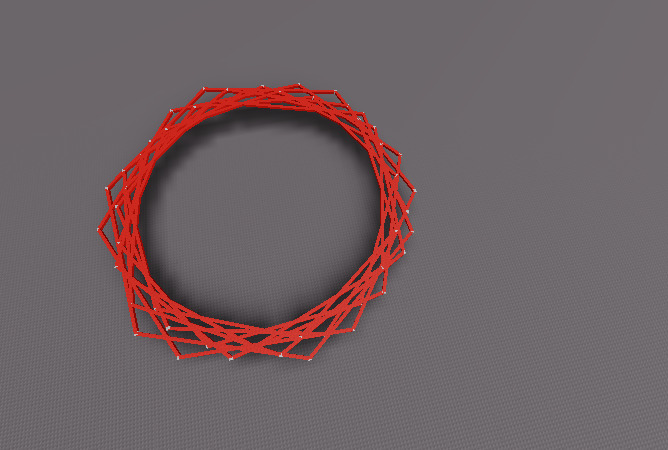# Is it a bug or i messed up something with CFrames and magnitudes?

I’m trying to connect all points with their next point in table but it gives me wrong magnitude and even if i created my own function for magnitude but it’s still returns me same numbers and it don’t makes any senseAlso CFrames are not working like they supposed to as you can see on the picture
why?

equations worked before (weeks ago)and everything is in it’s own order like it supposed to

2 Likes

Are you sure that your function for calculating the magnitude is correct.

``````local dist = magnitude(point,nextPoint)
``````

Just incase the correct implementation for getting the distance between two points should be

``````local dist = (point - nextPoint).Magnitude
``````100%
sure that’s working

That is not right at all.
First off is there any reason why you flip the sign while negative.
Second off, you cant slip up the equation into multiple sqrt roots, that breaks algebra.

The correct way is explained above using the Roblox method for it, or if you want to make your own function then you can just do…

``````function magnitude(a,b)
local o = a.x - b.x
local o2 = a.y - b.y
local o3 = a.z - b.z
return math.sqrt(o*o+o2*o2+o3*o3)
end
``````
1 Like

the problem with that is i used roblox magnitude also and it was giving me same resultsIts quite difficult to see since you draw so many iterations. But that looks correct to me…

That looks like it is connecting points correctly, just the wrong points. Which makes sense, since you’re using the index of a table `points` as your angle.

```
like this
```

so it shows up

``````like this
``````

no it’s not what i want to achieve

`a` is wrong.

You’re doing `a = 1, a = 2, ... , a = #points` because of your loop. I’m assuming you think this means “1 degree”, “2 degrees”, etc.

But `math.cos` and `sin` takes an angle in radians, not degrees. That means after `a=4` you’re already wrapped around a full rotation.

Instead of `math.cos(a)` you should do something like

``````local angle = 2 * math.pi * a / #points -- smoothly spread points around the circle
local x = r * math.cos(angle)
local z = r * math.sin(angle)
``````